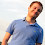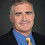## Tuesday, June 02, 2009

### A Kolmogorov Complexity Proof of the Lovász Local Lemma

Robin Moser gave one of the best STOC talks ever in his award-winning paper A Constructive Proof of the Lovász Local Lemma. Not only an amazing result but also an incredibly inventive simple proof that he came up with while preparing the talk.

Here is a sketch of Moser's proof written as a Kolmogorov complexity argument. This is not the full Lovász Local Lemma but captures the main principle.

Theorem: Suppose we have a k-CNF formula φ with n variables and m clauses and each clause shares a variable with at most r other clauses. Then there is a constant d such that if r < 2k-d then φ is satisfiable. Moreover we can find that assignment in time polynomial in m and n.

Here's the algorithm:
Solve(φ)
Pick a random assignment of φ
While there is an unsatisfiable clause C
Fix(C)
Fix(C)
Replace the variables of C with new random values
While there is clause D that shares a variable with C that is not satisfied
Fix(D)

Assume Fix(C) always terminates. Every clause that was satisfied before we called Fix(C) will still remain satisfied and C will also now be satisfied. So Solve makes at most m calls to Fix.

We need to show all the Fix(C) terminate. Suppose the algorithm makes s Fix calls including all the recursive ones. We will show s is bounded and thus the algorithm terminates.

Fix a Kolmogorov random string x of length n+sk (random relative to φ, k, s, r, m and n) and assume the algorithm uses the first n bits as the initial assignment and k bits each to replace the variables in each Fix call.
If we know which clause is being fixed, we know the clause is violated so we know all the bits of this clause and thus we learn k bits of x. We then replace those bits with another part of x.

So we can describe x by the list of clauses we fix plus the remaining n bits of the final assignment. We can describe the C such that Fix(C) is called by Solve by m log m bits and the remaining fixed clauses by log r + O(1) bits because either it is one of r clauses that intersects the previous clause or we indicate the end of a recursive call (keeping track of the recursion stack).

So we must have m log m + s(log r+O(1))+n ≥ n+sk or s(k-log r-O(1)) ≤ m log m.

To show s is bounded, we need k-log r-O(1) to be positive or r<2k-d for some constant d.

Note this in fact shows s = O(m log m) so the algorithm runs in polynomial time. We choose the x randomly which with high probability will be Kolmogorovly random. QED

In the talk, Moser gives the bound r<2 k-3 and in follow-up work with Gábor Tardos shows r<2 k/e (e = 2.71…) which is the best that comes out of the original Lovász Local Lemma.

1.Very nice! I'm sorry to have missed this talk (and this STOC).

2."Assume Fix(C) always terminates. Every clause that was satisfied before we called Fix(C) will still remain sastisfied and C will also now be satisfied."

Why will each clause that is satisfied remain satisfied? If there are two clauses, X and Y, that overlap in a variable, then if Y's assignment is changed so that it is satisfied, then now it is possible that X is no longer satisfied. What am I missing?

3.If there are two clauses, X and Y, that overlap in a variable, then if Y's assignment is changed so that it is satisfied, then now it is possible that X is no longer satisfied.I think the while loop in Fix() iterates over C also; i.e., it's not "While there is a clause D neq C that shares ..."

1.Fix(C)
Replace the variables of C with new random values
While there is clause D that shares a variable with C that is not satisfied
Fix(D)

It is in the second part: if to clauses X and Y that overlap then Y will be in one of the D that will be fixed. Assuming Fix(C) terminate the previously satisfied clauses remain satisfied.

4.What is an example of a formula with few satisfying assignments that satisfies the hypothesis of the lemma? I.e., a formula where you would need this algorithm because picking a random assignment would not work?

5.Great description of a great technique. This is much easier than the usual inductive proofs. To me the really brilliant point is the picking of a specific Kolmogorov/Chaiten at the start and not having to deal with randomness in the insides of the argument.

6.If I'm not mistaken:

Before: We had a nonconstructive existence proof.
After: We have a nonconstructive proof-of-correctness for an explicit construction.

We push the nonconstructiveness one level down. Is it possible to get rid of it completely?

7.Response to last anon.

LLL was non-constructive only in the sense of efficiency. The new proof gives an efficient (poly-time) randomized algorithm as a byproduct.

8.Anon2: you are missing the fact that Fix() terminates. If the new assignment to Y un-satisfies X, Y will call Fix() on X. This might look like an infinite recursion, but the proof shows it is not.

This one is definitely from the book. Very beautiful!

9.What is an example of a formula with few satisfying assignments that satisfies the hypothesis of the lemma?...

(avb)^(av~b)^(cvd)^(cv~d)^...
which you can generalize to kcnfs.

10.We can describe the C such that Fix(C) is called by Solve by m log m bits

Use a bitstring instead of a list and it only takes m bits, so s = O(m).

11.Nice post. But I never understand why such arguments are said to use "Kolmogorov complexity". It looks like straight information theory to me.

12.Moser's proof reminds me of the Chronogram Method in Data Structure Lower Bounds. Another coding argument that gives a lower bound, in a similar way.

13.Presumably you meant "at most s" instead of "at least s"?

1.I changed it to be just "s".

14.Can you please clarify how the constant d becomes 3?

1.It's not that simple. Best to read the paper.

15.I know this is an old post, but I'm still missing part of the argument, namely the running time bound.

For some sources of randomness the algorithm will finish sooner, for some later -- this makes thinking about s as universal number for every x a bit vague. My understanding is that one needs to bound the expectation of the running time -- is it somehow hidden in the assumption that x is Kolmogorov random string? Or is more work needed?

I also noticed that the paper by Moser and Tardos use somewhat different algorithm, one that avoids recursion.
Is it just a presentation detail, or do the two algorithms have different running times? Or is this the version
from the talk as opposed to the paper?